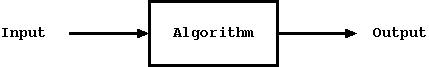## CodingBison

### Introduction

An algorithm is a computational procedure or a step by step mechanism to solve a problem. Algorithms are used so widely that they hardly need any introduction! They are used in all aspects of applications like search, graph, networking, security, etc. Typically, we would have an input data and we would like to solve a problem related to the input data -- the solution could be the output data. As an example, we may have a list of numbers (input data) and we would like to sort them. The result can be another list (output data) that contains these numbers, but in a sorted manner. Thus, we can see an algorithm as a box, where we feed input data and the algorithm returns the data in the desired form.Figure: Input/Output using Algorithm

When using an algorithm to solve a problem, there are three things to keep in mind. First is the algorithm itself -- basically, the step by step procedure that would help us solve the problem. Second is the data-structure that is needed to organize data. Depending upon how we organize data, we might end up using different algorithms. So, selecting the right data-structure is very important. Lastly, it is the implementation itself. Basically, having an implementation of algorithm using a programming language. In this module 'C' language is being used for implementing all the algorithms. Some of the modern programming languages offer a lot of in-built data structures and algorithms.

Given a problem, there could be more than one way to achieve the desired output for the same input and different approaches can very well use a different algorithm. When selecting the right algorithm, there are two important criteria. First is the running time (time-complexity) that measures the total time taken to finish the execution. Second is the extra space (space-complexity) it takes to finish the execution. With space (memory) becoming cheaper and cheaper by the year, people usually don't mind trading space with time in order to design an efficient algorithm. Accordingly, time complexity is given more weight over space complexity, while calculating the efficiency of an algorithm.

This module presents a handful of algorithms n C and focuses on sorting, search, and graph. For sorting, the module presents various algorithms like bubble-sort, selection-sort, quick-sort, etc. Equally important, it also presents various sorting algorithms that run in the linear time. In addition to sorting, the module also presents various graph algorithms like depth-first search, breadth-first search, minimum spanning trees, Dijkstra's algorithm, etc.

### Analysis of an Algorithm

You might be thinking, how do we analyze an Algorithm? Well, there are usually two factors involved in analyzing an algorithm: correctness and time-complexity. If an algorithm requires a lot of space, then we can throw in space-complexity as well.

Correctness of an algorithm means that its behavior should be as expected. In other words, For a given input the output should be as expected. This should hold true for all the cases and for all sizes of input.

For time-complexity (running time), we can look a the pseudo-code (or an actual code, if that is available) and analyze the compute operations for the algorithm. Big O notation is used to calculate the time-complexity of the algorithm. We will see how to calculate the running time using big O notation in the further sections while analyzing the algorithms.

Here are some of the commonly encountered Big O time-complexity types.

Table: Common Time-Complexity Values in Big O Notation
Time-ComplexityValueDescription
O(1)Constant timeTime taken by the algorithm is constant and does not change with the size of the data set
O(logN) or O(NlogN)Logarithmic timeTime taken increases at logN (or NlogN) rate
O(N)Linear timeTime taken increases linearly as the data size increases
O(N^2)Quadratic timeTime taken increases as square of the data size

In terms of time-complexity calculation, we need to calculate the time taken by an algorithm in three cases: (a) worst case -- when the given input data set require the algorithm to do maximum number of computations, (b) best case -- when the given input data set require the algorithm to do minimum number of computations, and (c) average case -- when the given input data set require the algorithm to do average number of computations